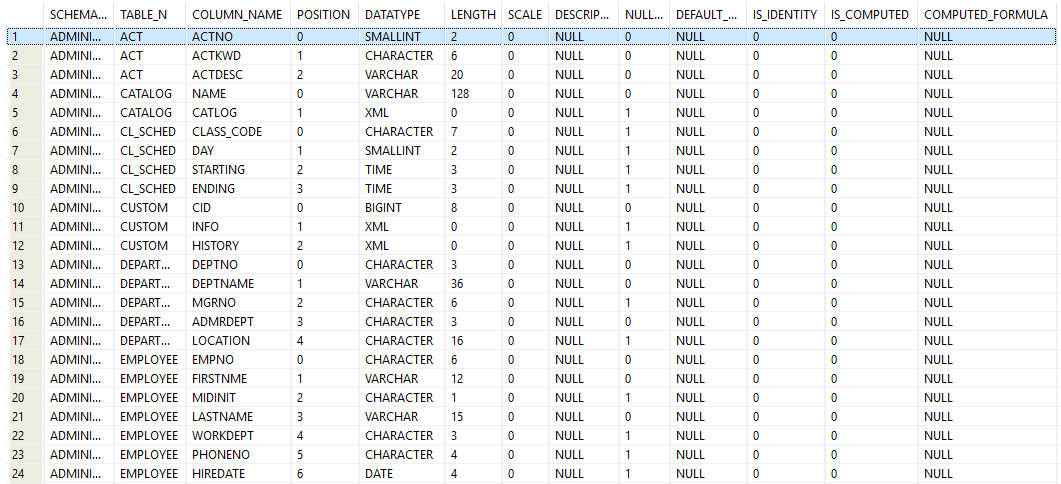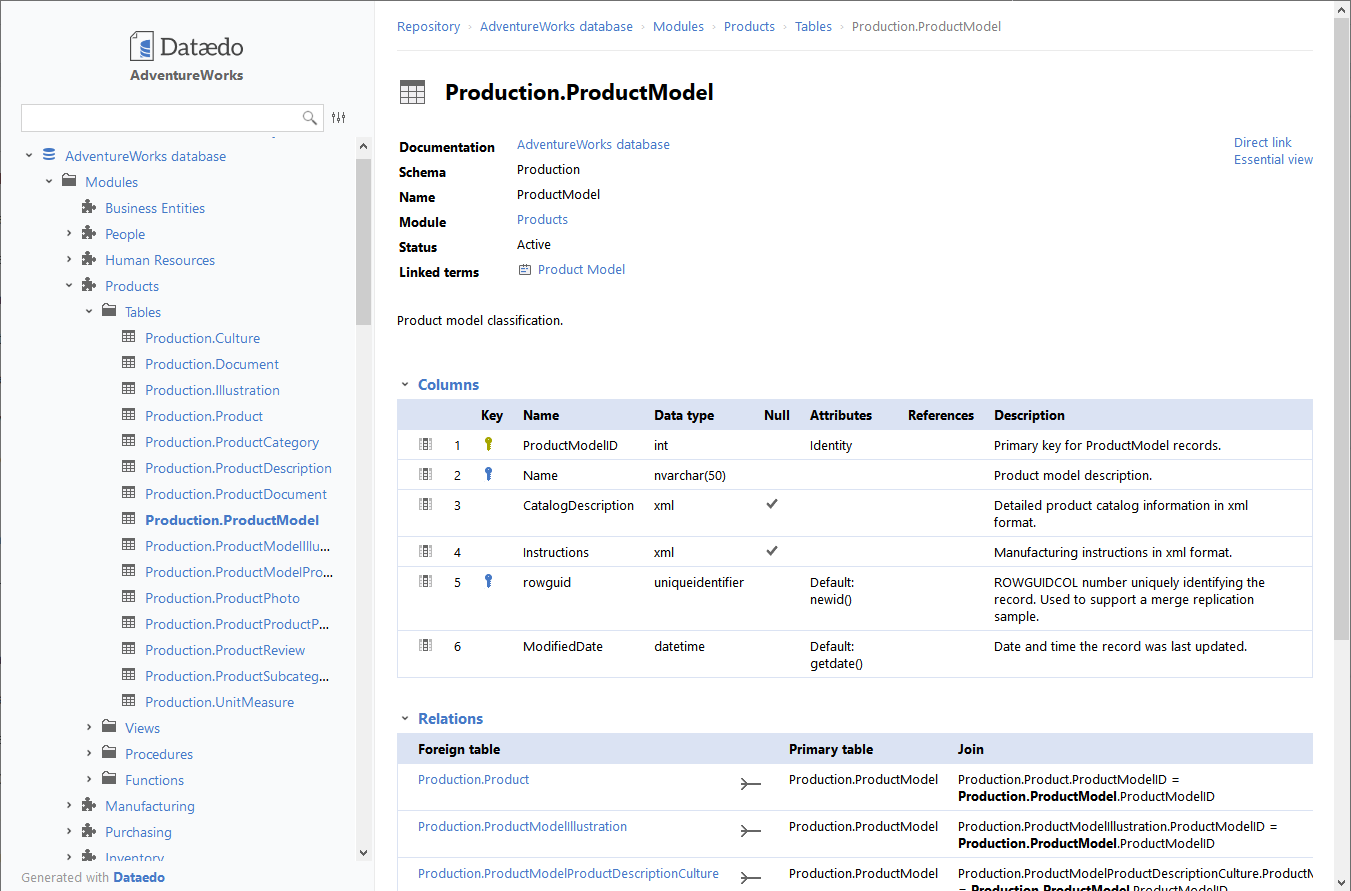## List table columns in Db2 database

Article for:

Query below lists all table columns in a database.

## Query

``````Select c.tabschema as schema_name,
c.tabname as table_name,
c.colname as column_name,
c.colno as position,
c.typename as data_type,
c.length,
c.scale,
c.remarks as description,
case when  c.nulls = 'Y' then 1 else 0 end as nullable,
default as default_value,
case when c.identity ='Y' then 1 else 0 end as is_identity,
case when c.generated ='' then 0 else 1 end as  is_computed,
c.text as computed_formula
from syscat.columns c
inner join syscat.tables t on
t.tabschema = c.tabschema and t.tabname = c.tabname
where t.type = 'T'
order by schema_name,
table_name
``````

## Columns

• schema_name - schema name
• view_name - table name
• column_name - column name
• position - number of this column in the table (starting with 0)
• data_type - name of the data typ
• length - maximum length of the data; 0 for distinct types.
• scale -
• scale if the column type is DECIMAL,
• number of digits of fractional seconds if the column type is TIMESTAMP,
• 0 otherwise
• description - description of column
• nullable - nullability attribute for the column
• is_identity - identity attribute for the column
• is_computed - computed (generated) attribute for the column
• computed_formula - the text of the computed column expression

## Rows

• One row represents one table column
• Scope of rows: all columns in all tables in a database
• Ordered by schema, table name, column id

## Sample results## You could also get this

Get this interactive HTML data dictionary in minutes with Dataedo.See live HTML data dictionary sample